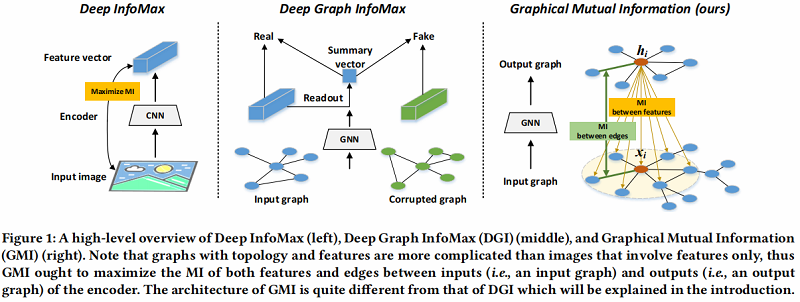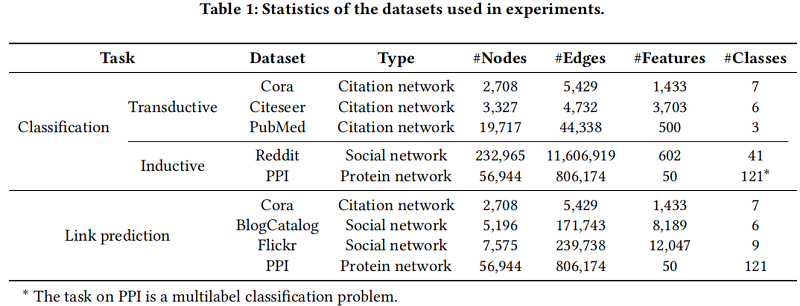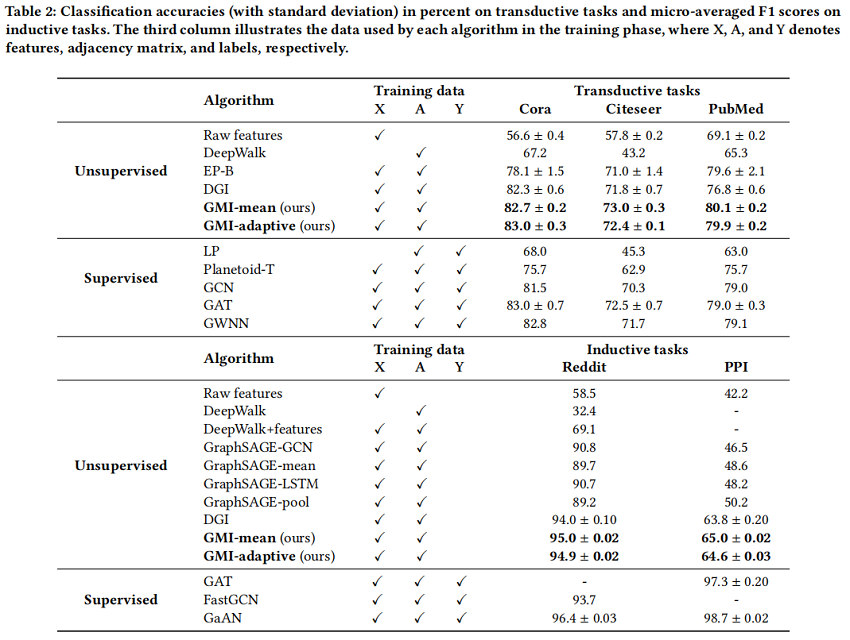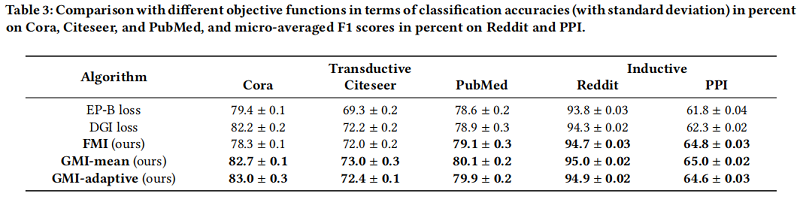# 1 Introduction

Deep Graph Infomax (DGI) ，通过最大化图级别表示向量和隐藏表示互信息之间的互信息【全局和局部信息之间的互信息】，来区分 Positive graph 和 Negative graph 。其存在的问题是：获取图级别表示的 Readout 函数常常是单设的，但是 Readout 的单射性质会受到参数训练方式的影响，这表明 Readout 函数在某些情况下会变成非单射。当 Readout 函数非单射时，图表示中包含的输入图信息将随着输入图的大小增大而减小【一对多造成】。

本文提出了一种直接的方法来考虑图结构方面的 $\text{MI}$，而不使用任何 Readout 函数和 corruption function作者通过比较编码器的输入（即由输入邻域组成的子图）和输出（即每个节点的隐藏表示），直接推导出 $\text{MI}$。[ 改进 ]作者理论推导表明，直接导出的 $\text{MI}$ 可以分解为每个邻居特征和隐藏向量之间的局部 $\text{MI}$ 的加权和。这样，我们对输入特征进行了分解，使 $\text{MI}$ 计算易于处理。此外，如果我们调整权值，这种形式的 $\text{MI}$ 可以很容易地满足对称性质。由于上述 $\text{MI}$ 主要是在节点特征级别上测量的，作者称之为特征互信息（FMI）

关于上述提到的 $\text{FMI}$ ，存在着两个问题：

• 组合的权重仍然未知；
• 没有考虑到拓扑结构；

为解决这两个问题，作者定义了基于 $\text{FMI}$ 提出了 Graphical Mutual Information（GMI），GMI 将 $\text{FMI}$ 中的权重设置为表示空间中每个邻居和目标节点之间的距离。为了保留拓扑信息，GMI 通过一个额外的互信息项进一步将这些权值与输入的边特征相关联。

# 2 Graphical mutual information:definition and maximization

承接 Introduction 中提到的 [ 改进 ] ，编码过程可以在节点级重写。

作者将节点 $i$ 的 $\boldsymbol{X}_{i}$ 和 $\boldsymbol{A}_{i}$ 分别定义为其邻居的特征矩阵和对应邻接矩阵特别地，当编码器 $f$ 是 $l$ 层的 GNN 时， $\boldsymbol{X}_{i}$ 由 $v_{i}$ 的所有 $k \leq l$ $\text{hop}$ 邻居组成，显然还可以进一步在邻接矩阵中添加自环，那么它则会包含节点 $i$ 本身信息。图中节点编码过程: $\boldsymbol{h}_{i}=f\left(\mathcal{G}_{i}\right)=f\left(\boldsymbol{X}_{i}, \boldsymbol{A}_{i}\right)$ 。

根据 Deep InfoMax（DIM）的思想，应最大化每个节点表示  $\boldsymbol{h}_{i}$  和  $\mathcal{G}_{i}$  之间的 $\text{MI}$（即 $I\left(\boldsymbol{h}_{i} ; \mathcal{G}_{i}\right)$ ）。但并没有一个较好的方法定义  $I\left(\boldsymbol{h}_{i} ; \mathcal{G}_{i}\right)$ ，原因是：

• $\text{MI}$ 应该具有平移不变性，即：如果 $\mathcal{G}_{i}$ 和 $\mathcal{G}_{i}^{\prime}$ 同构，那么 $I\left(\boldsymbol{h}_{i} ; \mathcal{G}_{i}\right)=I\left(\boldsymbol{h}_{i} ; \mathcal{G}_{i}^{\prime}\right)$ 。
• 如果采用 MINE 方法进行 $\text{ML}$ 计算，那么 MINE 中的判别器只接受固定大小的输入。但这对于  $\mathcal{G}_{i}$  是不可行的，因为不同的  $\mathcal{G}_{i}$  通常包含不同数量的邻居节点，因此具有不同的大小。

## 2.1 Feature Mutual Information

将 $\boldsymbol{X}_{i}$  的经验概率分布表示为  $p\left(\boldsymbol{X}_{i}\right)$， $\boldsymbol{h}_{i}$ 的概率分布表示为  $p\left(\boldsymbol{h}_{i}\right)$  ，联合分布用  $p\left(\boldsymbol{h}_{i}, \boldsymbol{X}_{i}\right)$ 表示。 根据信息论，$\boldsymbol{h}_{i}$ 和  $\boldsymbol{X}_{i}$  之间的 $\text{MI}$ 可以定义为：

${\large I\left(\boldsymbol{h}_{i} ; \boldsymbol{X}_{i}\right)=\int_{\mathcal{H}} \int_{\mathcal{X}} p\left(\boldsymbol{h}_{i}, \boldsymbol{X}_{i}\right) \log \frac{p\left(\boldsymbol{h}_{i}, \boldsymbol{X}_{i}\right)}{p\left(\boldsymbol{h}_{i}\right) p\left(\boldsymbol{X}_{i}\right)} d \boldsymbol{h}_{i} d \boldsymbol{X}_{i}}\quad\quad\quad(1)$

以下将根据互信息分解定理计算 $I\left(\boldsymbol{h}_{i} ; \boldsymbol{X}_{i}\right)$。

Theorem 1 (Mutual information decomposition). If the conditional probability  $p\left(\boldsymbol{h}_{i} \mid \boldsymbol{X}_{i}\right)$  is multiplicative, the global mutual information  $I\left(\boldsymbol{h}_{i} ; \boldsymbol{X}_{i}\right)$  defined in Eq. (1) can be decomposed as a weighted sum of local MIs, namely,

$I\left(\boldsymbol{h}_{i} ; \boldsymbol{X}_{i}\right)=\sum\limits _{j}^{i_{n}} w_{i j} I\left(\boldsymbol{h}_{i} ; \boldsymbol{x}_{j}\right)\quad\quad\quad\quad(2)$

其中：

• $x_{j}$  is the $j-th$ neighbor of node  $i$
• $i_{n}$  is the number of all elements in  $X_{i}$
• the weight  $w_{i j}$  satisfies  $\frac{1}{i_{n}} \leq w_{i j} \leq 1$  for each  $j$

为了证明 Theorem 1 ，引入两个 lemmas 和一个 definition

Lemma 1. For any random variables $X$, $Y$, and $Z$, we have

$I(X, Y ; Z) \quad \geq \quad I(X ; Z)\qquad \qquad (3)$

Lemma 1 证明：

$\begin{array}{l}I(X, Y ; Z)-I(X ; Z)\\ =\iiint_{X Y Z} p(X, Y, Z) \log \frac{p(X, Y, Z)}{p(X, Y) p(Z)} d X d Y d Z-\iint_{X Z} p(X, Z) \log \frac{p(X, Z)}{p(X) p(Z)} d X d Z\\ =\iiint_{X Y Z} p(X, Y, Z) \log \frac{p(X, Y, Z)}{p(X, Y) p(Z)} d X d Y d Z-\iiint_{X Y Z} p(X, Y, Z) \log \frac{p(X, Z)}{p(X) p(Z)} d X d Y d Z\\ =\iiint_{XYZ} p(X, Y, Z) \log \frac{p(X, Y, Z)}{p(Y \mid X) p(X, Z)} d X d Y d Z\\ =\iiint_{XYZ} p(Y, Z \mid X) p(X) \log \frac{p(Y, Z \mid X)}{p(Y \mid X) p(Z \mid X)} d X d Y d Z\\ =I(Y ; Z \mid X) \geq 0 \end{array}$

因此，$I(X,Y;Z) \ge I(X;Z)$。

Definition 1. The conditional probability $p\left(h \mid X_{1}, \cdots, X_{n}\right)$ is called multiplicative if it can be written as a product

$p\left(h \mid X_{1}, \cdots, X_{n}\right)=r_{1}\left(h, X_{1}\right) \cdots r_{n}\left(h, X_{n}\right)\quad\quad\quad\quad(4)$

其中 $r_1, · · · ,r_n$ 是 appropriate functions 。

Lemma 2. If $p\left(h \mid X_{1}, \cdots, X_{n}\right)$ is multiplicative, then we have

$I(X ; Z)+I(Y ; Z) \geq I(X, Y ; Z)\quad\quad\quad(5)$

Theorem 1 证明：

根据 Lemma 1 ，对于任何一个 $j$ ：

$I\left(\boldsymbol{h}_{i} ; \boldsymbol{X}_{i}\right)=I\left(\boldsymbol{h}_{i} ; \boldsymbol{x}_{1}, \cdots, \boldsymbol{x}_{i_{n}}\right) \geq I\left(\boldsymbol{h}_{i} ; \boldsymbol{x}_{j}\right)\quad\quad\quad(6)$

有：

$I\left(\boldsymbol{h}_{i} ; \boldsymbol{X}_{i}\right)=\sum\limits \frac{1}{i_{n}} I\left(\boldsymbol{h}_{i} ; \boldsymbol{X}_{i}\right) \geq \sum \limits \frac{1}{i_{n}} I\left(\boldsymbol{h}_{i} ; \boldsymbol{x}_{j}\right)\quad\quad\quad(7)$

根据 Lemma 2 ，得到：

$I\left(\boldsymbol{h}_{i} ; \boldsymbol{X}_{i}\right) \leq \sum\limits I\left(\boldsymbol{h}_{i} ; \boldsymbol{x}_{j}\right)\quad\quad\quad(8)$

根据 $\text{Eq.7}$ 和 $\text{Eq.8}$ ：

$\sum\limits \frac{1}{i_{n}} I\left(\boldsymbol{h}_{i} ; \boldsymbol{x}_{j}\right) \leq I\left(\boldsymbol{h}_{i} ; \boldsymbol{X}_{i}\right) \leq \sum\limits I\left(\boldsymbol{h}_{i} ; \boldsymbol{x}_{j}\right)\quad\quad \quad(9)$

因为  $I\left(\boldsymbol{h}_{i} ; \boldsymbol{x}_{j}\right) \geq 0$  ，必须存在权重  $\frac{1}{i_{n}} \leq w_{i j} \leq 1$。 当设置  $w_{i j}=I\left(\boldsymbol{h}_{i} ; \boldsymbol{X}_{i}\right) / \sum I\left(\boldsymbol{h}_{i} ; \boldsymbol{x}_{j}\right)$  时，我们将实现 $\text{Eq.2}$，同时确保  $\frac{1}{i_{n}} \leq w_{i j} \leq 1$，进而证明了定理1。

此外，可以调整权值，以反映输入图的同构变换。例如，如果 $\boldsymbol{X}_{i}$ 只包含节点 $i$ 的 $1-h o p$ 邻居，则将所有权重设置为相同， 将导致不同顺序的输入节点产生相同的 $\mathrm{MI}$。

## 2.2 Topology-Aware Mutual Information

受 Theorem 1 的启发，试图从图的 拓扑 结构构造可训练的权值 $w_{ij}$。

Definition 2 (Graphical mutuak mutual information). The MI between the hidden vector  $\boldsymbol{h}_{i}$  and its support graph  $\mathcal{G}_{i}=\left(\boldsymbol{X}_{i}, \boldsymbol{A}_{i}\right)$  is defined as

$\begin{array}{c} I\left(\boldsymbol{h}_{i} ; \mathcal{G}_{i}\right):=\sum\limits _{j}^{i_{n}} w_{i j} I\left(\boldsymbol{h}_{i} ; \boldsymbol{x}_{j}\right)+I\left(w_{i j} ; \boldsymbol{a}_{i j}\right), \\ \text { with } w_{i j}=\sigma\left(\boldsymbol{h}_{i}^{\mathrm{T}} \boldsymbol{h}_{j}\right) \end{array}\quad\quad \quad(10)$

其中 $\boldsymbol{x}_{j}$ 和 $i_{n}$ 的定义与 Theorem  1 相同，$\boldsymbol{a}_{i j}$ 是邻接矩阵 $A$ 中的边权值，$\sigma(\cdot)$ 是一个 $\text{sigmoid}$ 函数

$Eq.10$ 中第一项的  $w_{i j}$  衡量了一个局部 $\text{MI}$ 对全局 $\text{MI}$ 的贡献，即  $w_{i j}= \sigma\left(\boldsymbol{h}_{i}^{T} \boldsymbol{h}_{j}\right)$。同时，$I\left(w_{i j} ; \boldsymbol{a}_{i j}\right. )$ 最大化  $w_{i j}$  和输入图的边权重 $\boldsymbol{a}_{i j}$ 之间的 $\text{MI}$ ，以强制  $w_{i j}$ 符合拓扑关系。

## 2.3 Maximization of GMI

本文为了有效性和效率，选用 JSD 估计器，因为 infoNCE 估计器对负面采样策略敏感，因此可能成为固定可用内存的大规模数据集的瓶颈。相反，JSD 估计器对负抽样策略的不敏感性及其在许多任务上的良好性能使其更适合我们的任务。

接着作者通过下式计算 Eq.10 中的第一项：

$I\left(\boldsymbol{h}_{i} ; \boldsymbol{x}_{j}\right)=-s p\left(-\mathcal{D}_{w}\left(\boldsymbol{h}_{i}, \boldsymbol{x}_{j}\right)\right)-\mathbb{E}_{\tilde{\mathbb{P}}}\left[\operatorname{sp}\left(\mathcal{D}_{w}\left(\boldsymbol{h}_{i}, \boldsymbol{x}_{j}^{\prime}\right)\right)\right]\quad\quad\quad(11)$

其中

• $\mathcal{D}_{w}: D \times D^{\prime} \rightarrow \mathbb{R}$ 是由参数为 $w$ 的神经网络构建的判别器；
• $x^{\prime}{ }_{j}$ 是来自 $\tilde{\mathbb{P}}=\mathbb{P}$ 的负样本；
• $s p(x)=\log \left(1+e^{x}\right)$，即soft-plus function；

本文通过计算交叉熵而不是使用 JSD 估计器使  $I\left(w_{i j} ; \boldsymbol{\alpha}_{i j}\right)$  最大化：

$I\left(w_{i j} ; \boldsymbol{a}_{i j}\right)=\boldsymbol{a}_{i j} \log w_{i j}+\left(1-\boldsymbol{a}_{i j}\right) \log \left(1-w_{i j}\right)\quad\quad\quad(12)$

# 3 Experiments2022-03-26 创建文章
2022-06-13 二次阅读